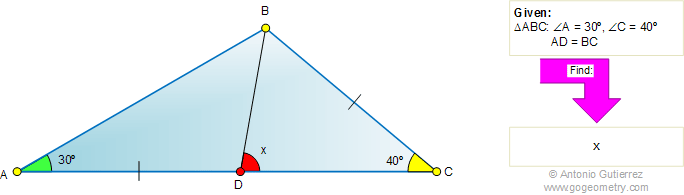# Geometry Problem 423: Triangle, 30, 40 degree, Angle, Congruence

 The figure shows a triangle ABC with angles A and C equal to 30 and 40 degrees, respectively. If AD = BC, find the measure of angle BDC.Home | Search | Geometry | Problems | All Problems | 421-430 | Email | Solution / comment | By Antonio Gutierrez